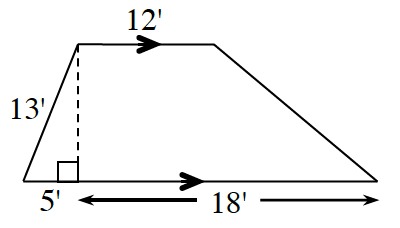### Home > GC > Chapter 2 > Lesson 2.3.3 > Problem2-120

2-120.

Find the area of the trapezoid at right. What strategies did you use?

Look at the Math Notes box in Lesson 2.2.4 for help.

$\begin{array}{r} 5^2 + c^2 = 13^2 \\ -25 \qquad -25 \end{array}$

$\text{Area of a trapezoid }=\frac{1}{2}(b_{1}+b{_{2}})h$